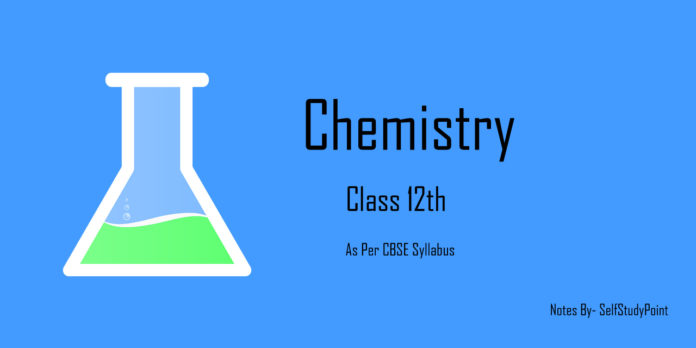# Ideal and Non-Ideal Solutions:

Liquid-liquid solutions can be classified as ideal and non-ideal on the basis of certain properties.

## Ideal Solution:

• Obeys the Roult’s Law over the entire range of concentration.
• ΔHmixing = 0
• ΔVmixing = 0
• Example: solution of n-hexane and n-heptane, a solution of bromoethane or chloroethane.

It can be summarized as: If a Solution formed by mixing the two components A and B, in which intermolecular force of attraction between A and B (A‒B) is nearly equal to intermolecular force of attraction between pure components (A‒A and B‒B) then no heat would be evolved or absorbed in forming the solution. Also, volume of the solution will be equal to the total volume of the individual component taken to form the solution.

## Non-Ideal Solution:

• Does not obey the Roult’s Law over the entire range of concentration.
• ΔHmixing ≠ 0
• ΔVmixing ≠ 0
• Example: Solution of chloroform and acetone

It can be summarized as If a Solution formed by mixing the two components A and B, in which intermolecular force of attraction between A and B (A‒B) is not equal to the intermolecular force of attraction between pure components (A‒A and B‒B). This new interaction (A‒B) is either less than or more than the interaction of the pure components (A‒A and B‒B). This leads to the positive or negative deviations from Roult’s Law.